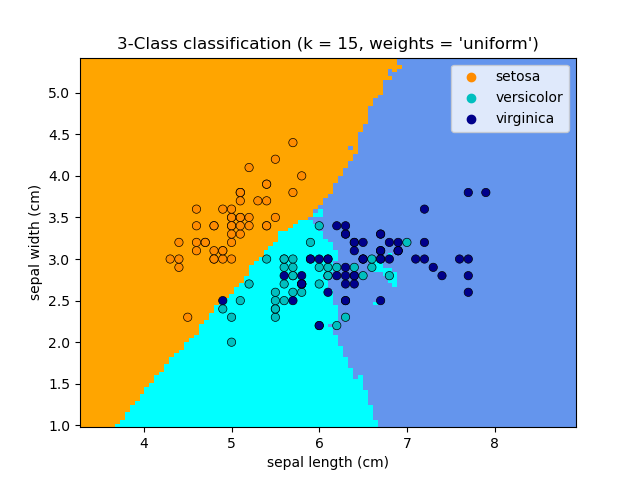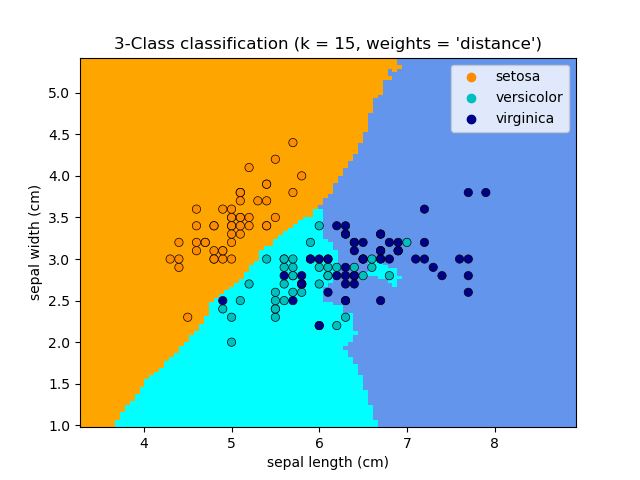# Nearest Neighbors Classification¶

Sample usage of Nearest Neighbors classification. It will plot the decision boundaries for each class.

••import matplotlib.pyplot as plt
import seaborn as sns
from matplotlib.colors import ListedColormap
from sklearn import neighbors, datasets
from sklearn.inspection import DecisionBoundaryDisplay

n_neighbors = 15

# import some data to play with

# we only take the first two features. We could avoid this ugly
# slicing by using a two-dim dataset
X = iris.data[:, :2]
y = iris.target

# Create color maps
cmap_light = ListedColormap(["orange", "cyan", "cornflowerblue"])
cmap_bold = ["darkorange", "c", "darkblue"]

for weights in ["uniform", "distance"]:
# we create an instance of Neighbours Classifier and fit the data.
clf = neighbors.KNeighborsClassifier(n_neighbors, weights=weights)
clf.fit(X, y)

_, ax = plt.subplots()
DecisionBoundaryDisplay.from_estimator(
clf,
X,
cmap=cmap_light,
ax=ax,
response_method="predict",
plot_method="pcolormesh",
xlabel=iris.feature_names,
ylabel=iris.feature_names,
)

# Plot also the training points
sns.scatterplot(
x=X[:, 0],
y=X[:, 1],
hue=iris.target_names[y],
palette=cmap_bold,
alpha=1.0,
edgecolor="black",
)
plt.title(
"3-Class classification (k = %i, weights = '%s')" % (n_neighbors, weights)
)

plt.show()


Total running time of the script: ( 0 minutes 0.491 seconds)

Gallery generated by Sphinx-Gallery